An infinite number of electric charges each equal to 5 nano-coulomb (magnitude) are placed along x-axis at x = 1 cm, x = 2 cm, x = 4 cm, x = 8 cm ………. and so on. In the setup if the consecutive charges have opposite sign, then the electric field in Newton/Coulomb at x = 0 is $\left(\frac{1}{4\pi {\epsilon }_{0}}=9×{10}^{9}N-{m}^{2}/{c}^{2}\right)$

(1) 12 × 104

(2) 24 × 104

(3) 36 × 104

(4) 48 × 104

Concept Questions :-

Electric field
High Yielding Test Series + Question Bank - NEET 2020

Difficulty Level:

A small sphere carrying a charge ‘q’ is hanging in between two parallel plates by a string of length L. Time period of pendulum is T0. When parallel plates are charged, the time period changes to T. The ratio T/T0 is equal to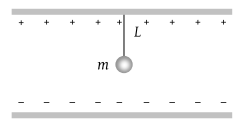(1) ${\left(\frac{g+\frac{qE}{m}}{g}\right)}^{1/2}$

(2) ${\left(\frac{g}{g+\frac{qE}{m}}\right)}^{3/2}$

(3) ${\left(\frac{g}{g+\frac{qE}{m}}\right)}^{1/2}$

(4) None of these

Concept Questions :-

Electric field
High Yielding Test Series + Question Bank - NEET 2020

Difficulty Level:

Three charges –q1, +q2 and –q3 are placed as shown in the figure. The x-component of the force on –q1 is proportional to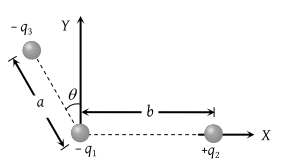(1) $\frac{{q}_{2}}{{b}^{2}}-\frac{{q}_{3}}{{a}^{2}}\mathrm{sin}\theta$

(2) $\frac{{q}_{2}}{{b}^{2}}-\frac{{q}_{3}}{{a}^{2}}\mathrm{cos}\theta$

(3) $\frac{{q}_{2}}{{b}^{2}}+\frac{{q}_{3}}{{a}^{2}}\mathrm{sin}\theta$

(4) $\frac{{q}_{2}}{{b}^{2}}+\frac{{q}_{3}}{{a}^{2}}\mathrm{cos}\theta$

Concept Questions :-

Electric field
High Yielding Test Series + Question Bank - NEET 2020

Difficulty Level:

Two-point charges +q and –q are held fixed at (–d, 0) and (d, 0) respectively of a (x, y) coordinate system. Then

(1) E at all points on the y-axis is along $\stackrel{^}{i}$

(2) The electric field $\stackrel{\to }{E}$ at all points on the x-axis has the same direction

(3) Dipole moment is 2qd directed along $\stackrel{^}{i}$

(4) Work has to be done in bringing a test charge from infinity to the origin

Concept Questions :-

Electric dipole
High Yielding Test Series + Question Bank - NEET 2020

Difficulty Level:

A point charge of 40 stat coulomb is placed 2 cm in front of an earthed metallic plane plate of large size. Then the force of attraction on the point charge is

(1) 100 dynes

(2) 160 dynes

(3) 1600 dynes

(4) 400 dynes

Concept Questions :-

Coulomb's law
High Yielding Test Series + Question Bank - NEET 2020

Difficulty Level:

Four charges equal to – Q are placed at the four corners of a square and a charge q is at its centre. If the system is in equilibrium the value of q is

1. $-\frac{Q}{4}\left(1+2\sqrt{2}\right)$

2. $\frac{Q}{4}\left(1+2\sqrt{2}\right)$

3. $-\frac{Q}{2}\left(1+2\sqrt{2}\right)$

4. $\frac{Q}{2}\left(1+2\sqrt{2}\right)$

Concept Questions :-

Coulomb's law
High Yielding Test Series + Question Bank - NEET 2020

Difficulty Level:

Which of the following graphs shows the variation of electric field E due to a hollow spherical conductor of radius R as a function of distance from the centre of the sphere

(1)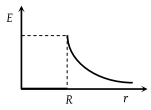(2)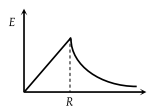(3)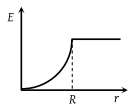(4)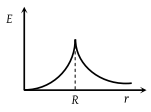Concept Questions :-

Electric field
High Yielding Test Series + Question Bank - NEET 2020

Difficulty Level:

The electric field due to a uniformly charged sphere of radius R as a function of the distance from its centre is represented graphically by

(1)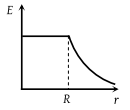(2)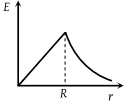(3)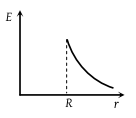(4)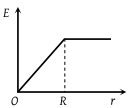Concept Questions :-

Electric field
High Yielding Test Series + Question Bank - NEET 2020

Difficulty Level:

Two concentric conducting thin spherical shells A and B having radii rA and rB (rB > rA) are charged to QA and $-{Q}_{B}\left(|{Q}_{B}|\text{\hspace{0.17em}}>\text{\hspace{0.17em}}|{Q}_{A}|\right)$. The electrical field at distance x from the common center is -

1.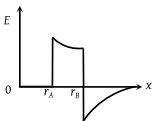2.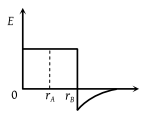3.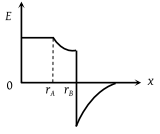4.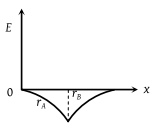Concept Questions :-

Electric field
High Yielding Test Series + Question Bank - NEET 2020

Difficulty Level:

Suppose the charge of a proton and an electron differ slightly. One of them is -e and the other is $\left(e+∆e\right)$. If the net of electrostatic force and gravitaional force between two hydrogen atoms placed at a distance d (much greater than atomic size) apart is zero,then $∆e$ is of the order [Given mass of hydrogen, ${m}_{h}$=1.67$×{10}^{-27}$ kg]

(a) ${10}^{-20}C$

(b)${10}^{-23}C$

(c) ${10}^{-37}C$

(d) ${10}^{-47}C$

Concept Questions :-

Coulomb's law